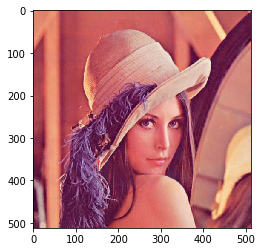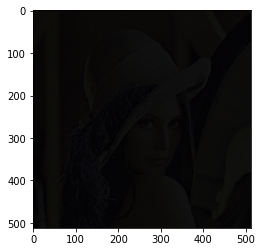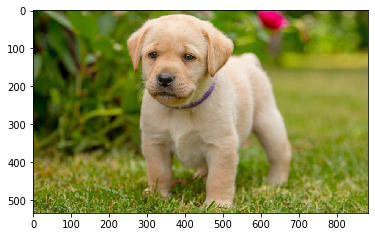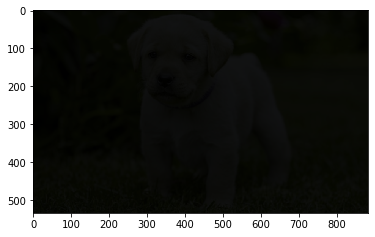# Mahotas – RGB to XYZ Conversion

In this article we will see how we can covert rgb image to xyz image in mahotas. An RGB image, sometimes referred to as a truecolor image, is stored in MATLAB as an m-by-n-by-3 data array that defines red, green, and blue color components for each individual pixel. Xyz is an additive color space based on how the eye intereprets stimulus from light. Unlike other additive rgb like Rgb, Xyz is a purely mathmatical space and the primary components are “imaginary”, meaning we can’t create the represented color in the physical by shining any sort of lights representing x, y, and z.

In this tutorial we will use “lena” image, below is the command to load it.

`mahotas.demos.load('lena')`

Below is the lena imageIn order to do this we will use `mahotas.colors.rgb2xyz`method

Syntax : mahotas.colors.rgb2xyz(img)

Argument :It takes image object as argument

Return : It returns image object

Below is the implementation

 `# importing required libraries ` `import` `mahotas ` `import` `mahotas.demos ` `from` `pylab ``import` `gray, imshow, show ` `import` `numpy as np ` `  `  `# loading iamge ` `img ``=` `mahotas.demos.load(``'lena'``) ` ` `  `  `  `# showing image ` `print``(``"Image"``) ` `imshow(img) ` `show() ` ` `  `# rgb to lab ` `new_img ``=` `mahotas.colors.rgb2xyz(img) ` ` `  `# showing new image ` `print``(``"New Image"``) ` `imshow(new_img) ` `show() `

Strong :

`Image``New Image`Another example

 `# importing required libraries ` `import` `mahotas ` `import` `numpy as np ` `import` `matplotlib.pyplot as plt ` `import` `os ` `  `  `# loading iamge ` `img ``=` `mahotas.imread(``'dog_image.png'``) ` `       `  `# fltering image ` `img ``=` `img[:, :, :``3``] ` ` `  `# showing image ` `print``(``"Image"``) ` `imshow(img) ` `show() ` ` `  `# rgb to lab ` `new_img ``=` `mahotas.colors.rgb2xyz(img) ` ` `  `# showing new image ` `print``(``"New Image"``) ` `imshow(new_img) ` `show() `

Strong :

`Image``New Image`My Personal Notes arrow_drop_upCheck out this Author's contributed articles.

If you like GeeksforGeeks and would like to contribute, you can also write an article using contribute.geeksforgeeks.org or mail your article to contribute@geeksforgeeks.org. See your article appearing on the GeeksforGeeks main page and help other Geeks.

Please Improve this article if you find anything incorrect by clicking on the "Improve Article" button below.

Article Tags :

Be the First to upvote.

Please write to us at contribute@geeksforgeeks.org to report any issue with the above content.